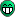# Cloning the axis of a histogram into the definition of another

Hi all,

I was wondering if ROOT allows for something like the following

``````
TH1D *h1d = new TH1D("h1d","h1d",10,0,10);

TAxis *oneD_Xaxis = h1d->GetXaxis();

TH2D *h2d = new TH2D("h2d","h2d",oneD_Xaxis->GetNbins(),oneD_Xaxis->GetXbins(),5,0,5);``````

which I would expect to be the same as

``TH2D *h2d = new TH2D("h2d","h2d",10,0,10,5,0,5);``

Thanks

Try:
std::cout << oneD_Xaxis->GetXbins()->GetSize() << std::endl;

I get ‘0’ ?

dashing, noWell, you’d better read about “fix” versus “variable” bin size histograms.So does this mean that the functionality I am looking for does not exist yet in ROOT?

If you mean fixed width versus variable bin width i understand the difference there, but I do not see how that applies to what I am saying here. In my first example ‘h1d’ I am using fixed bin widths.

For ‘h2d’ , and this is the subject of my question, I am attempting to combine the definition of a variable bin width histogram and a fixed bin width histogram. My real question is, can you use a constructor like that?

Found the answer to my problem, I will post the useful response here

``````TH1D *h1d = new TH1D("h1d","h1d",10,0,10);

TAxis *oneD_Xaxis = h1d->GetXaxis();

TH2D *h2d = new TH2D("h2d","h2d",oneD_Xaxis->GetNbins(),oneD_Xaxis->GetXbins()->GetArray(),5,0,5);``````

Try:
std::cout << oneD_Xaxis->GetXbins()->GetArray() << std::endl;